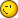# Cplua 0.8 Waitpen Bug

2 replies to this topic

### #1Guest_adrahil_*

•• Guests

Posted 28 November 2005 - 02:06 PM

Hi!
I've tried the new 0.8 version and found out that there is a bug when calling the waitpen function like this:

fullscreen(1)
require("draw")
showgraph()
draw.onbuffer()

repeat
px,py=waitpen()
draw.circle(px,py,1,1,1)
draw.update()
until testkey(K_EXE)

This code works perfectly on CPLua 0.73RC2, so there must have been a bug introduced somewhere....

Cheers,

### #2Orwell

Orwell

Casio Overlord

•• Members
•• 777 posts
• Gender:Male
• Location:Paris - France

• Calculators:
Casio AFX 1.02 / Casio ClassPad 300

Posted 28 November 2005 - 03:24 PM

Well thanks, but rather than opening a new topic for each bug you should just say it in the main topic of CPLua.I'll try to fix it as soon as possible.

### #3adrahil

Newbie

•• Members
•• 3 posts

Posted 28 November 2005 - 07:23 PM

Sorry, I didn't see that that topic was also for bugsIt's really a pity that this function is *dead* for the moment. I was experimenting with CPLua for a few days right now and it is quite nice. The only things it lacks for the moment are the I/O functions for inter-calculator communication, and for writing files...

The beginning of a small TicTacToe I wrote in CPLua:
```keypad(0)
fullscreen(1)
require("draw")
require("mat")
showgraph()
draw.onbuffer()

function redraw()
draw.clear()
draw.line(4,4,154,4,1,2)
draw.line(4,54,154,54,1)
draw.line(4,104,154,104,1)
draw.line(4,154,154,154,2)
draw.line(4,4,4,154,1,2)
draw.line(54,4,54,154,1,1)
draw.line(104,4,104,154,1,1)
draw.line(154,4,154,154,1,2)
draw.rect(4,176,82,204,1)
draw.text(12,185,"recommencer")
draw.update()
end

redraw()
player=0
-- since there is no *trivial* method to flush a matrix, I copy an empty matrix into a non-empty one...
zzx=mat.new(10)
zz=mat.new(zzx)

repeat
x,y=waitpen()

--new game...
if x>=4 and x<=82 and y>=176 and y<=204 then
zz=mat.new(zzx)
redraw()
end

if x>=4 and x<=154 and y>=4 and x<=154 then
xx=x-4-math.mod(x-4,50)
yy=y-4-math.mod(y-4,50)
if zz[xx/50+yy*3/50+1]==0 then
if player==0 then
draw.line(10+xx,10+yy,48+xx,48+yy,1)
draw.line(10+xx,48+yy,48+xx,10+yy,1)
player=1
zz[xx/50+yy*3/50+1]=1
elseif player==1 then
draw.circle(29+xx,29+yy,18,1)
player=0
zz[xx/50+yy*3/50+1]=2
end
end
draw.update
end
until testkey(E_EXE)```
By the way, the program doesn't die when you press on EXE because of the waitpen... either the waitpen works (0.73), or the testkey does (0.8). To kill it use the ESC key...

#### 0 user(s) are reading this topic

0 members, 0 guests, 0 anonymous users